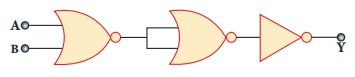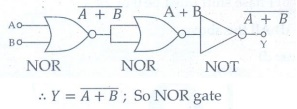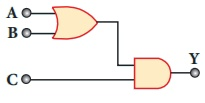# UNIT X: Semiconductor Electronics and Communication Systems - Online Test

Q1. If the input to the NOT gate is A = 1011, its output is
Explaination / Solution:

For NOT Gate output Y = Ᾱ

Here A = 1011 . Y =0100

Q2. Which one of the following represents forward bias diode?
Explaination / Solution:
No Explaination.

Q3. The given electrical network is equivalent toExplaination / Solution:Q4. The output of the following circuit is 1 when the input ABC isExplaination / Solution:

Y = (A+B)C

For Y = 1; C must be 1

The input ABC is 101

Y= (1 + 0).l = 1

Q5. The variation of frequency of carrier wave with respect to the amplitude of the modulating signal is called ------------------
Explaination / Solution:
No Explaination.

Q6. The frequency range of 3 MHz to 30 MHz is used for
Explaination / Solution:
No Explaination.

Q7. The specific characteristic of a common emitter amplifier is
Explaination / Solution:
No Explaination.

Q8. The electrical series circuit in digital form is
Explaination / Solution:
No Explaination.

Q9. The output transducer of the communication system converts the radio signal into _______
Explaination / Solution:
No Explaination.

Q10. The signal is affected by noise in a communication system
Explaination / Solution:
No Explaination.